### Algebraic Expressions - Solutions

CBSE Class –VII Mathematics

NCERT Solutions
Algebraic Expressions (Ex. 12.1)

Question 1. Get the algebraic expressions in the following cases using variables, constants and arithmetic operations:

1. Subtraction of $z$ from $y.$
2. One-half of the sum of numbers $x$ and $y.$
3. The number $z$ multiplied by itself.
4. One-fourth of the product of numbers $p$ and $q.$
5. Numbers $x$ and $y$ both squared and added.
6. Number 5 added to three times the product of $m$ and $n.$
7. Product of numbers $y$ and $z$ subtracted from 10.
8. Sum of numbers $a$ and $b$ subtracted from their product.

Answer: (i) $y-z$

(ii) $\frac{x+y}{2}$

(iii) ${z}^{2}$

(iv) $\frac{pq}{4}$

(v) ${x}^{2}+{y}^{2}$

(vi) $3mn+5$

(vii) $10-yz$

(viii) $ab-\left(a+b\right)$

Question 2.(i) Identify the terms and their factors in the following expressions, show the terms and factors by tree diagram:

(a) $x-3$

(b) $1+x+{x}^{2}$

(c) $y-{y}^{3}$

(d) $5x{y}^{2}+7{x}^{2}y$

(e) $-ab+2{b}^{2}-3{a}^{2}$

(ii) Identify the terms and factors in the expressions given below:

(a) $-4x+5$

(b) $-4x+5y$

(c) $5y+3{y}^{2}$

(d) $xy+2{x}^{2}{y}^{2}$

(e) $pq+q$

(f) $1.2ab-2.4b+3.6a$

(g) $\frac{3}{4}x+\frac{1}{4}$

(h) $0.1{p}^{2}+0.2{q}^{2}$

Answer: (i) (a) $x-3$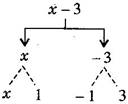(b) $1+x+{x}^{2}$

Expression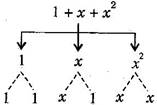(c) $y-{y}^{3}$

Expression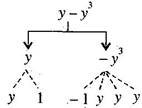(d) $5x{y}^{2}+7{x}^{2}y$

Expression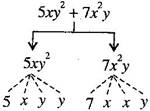(e) $-ab+2{b}^{2}-3{a}^{2}$

Expression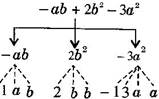(ii) (a) $-4x+5$

Terms: $-4x,5$

Factors:

(b) $-4x+5y$

Terms: $-4x,5y$

Factors:

(c) $5y+3{y}^{2}$

Terms: $5y,3{y}^{2}$

Factors:

(d) $xy+2{x}^{2}{y}^{2}$

Terms: $xy,2{x}^{2}{y}^{2}$

Factors:

(e) $pq+q$

Terms: $pq,q$

Factors:

(f) $1.2ab-2.4b+3.6a$

Terms: $1.2ab,-2.4b,3.6a$

Factors:

(g) $\frac{3}{4}x+\frac{1}{4}$

Terms: $\frac{3}{4}x,\frac{1}{4}$

Factors:

(h) $0.1{p}^{2}+0.2{q}^{2}$

Terms: $0.1{p}^{2},0.2{q}^{2}$

Factors:

Question 3.Identify the numerical coefficients of terms (other than constants) in the following expressions:

(i)$5-3{t}^{2}$

(ii)$1+t+{t}^{2}+{t}^{3}$

(iii)$x+2xy+3y$

(iv) $100m+1000n$

(v)$-{p}^{2}{q}^{2}+7pq$

(vi) $1.2a+0.8b$

(vii) $3.14{r}^{2}$

(viii) $2\left(l+b\right)$

(ix)  $0.1y+0.01{y}^{2}$

 S.No. Expression Terms Numerical Coefficient (i) 5−3t2$5-3{t}^{2}$ −3t2$-3{t}^{2}$ −3$-3$ (ii) 1+t+t2+t3$1+t+{t}^{2}+{t}^{3}$ t$t$ 1 t2${t}^{2}$ 1 t3${t}^{3}$ 1 (iii) x+2xy+3y$x+2xy+3y$ x$x$ 1 2xy$2xy$ 2 3y$3y$ 3 (iv) 100m+1000n$100m+1000n$ 100m$100m$ 100 1000n$1000n$ 1000 (v) −p2q2+7pq$-{p}^{2}{q}^{2}+7pq$ −p2q2$-{p}^{2}{q}^{2}$ −1$-1$ 7pq$7pq$ 7 (vi) 1.2a+0.8b$1.2a+0.8b$ 1.2a$1.2a$ 1.2 0.8b$0.8b$ 0.8 (vii) 3.14r2$3.14{r}^{2}$ 3.14r2$3.14{r}^{2}$ 3.14 (viii) 2(l+b)=2l+2b$2\left(l+b\right)=2l+2b$ 2l$2l$ 2 2b$2b$ 2 (ix) 0.1y+0.01y2$0.1y+0.01{y}^{2}$ 0.1y$0.1y$ 0.1 0.01y2$0.01{y}^{2}$ 0.01

Question 4.(a) Identify terms which contain $x$ and give the coefficient of $x.$

(i) ${y}^{2}x+y$

(ii) $13{y}^{2}-8yx$

(iii) $x+y+2$

(iv) $5+z+zx$

(v) $1+x+xy$

(vi) $12x{y}^{2}+25$

(vii) $7x+x{y}^{2}$

(b) Identify terms which contain ${y}^{2}$ and give the coefficient of ${y}^{2}.$

(i) $8-x{y}^{2}$

(ii) $5{y}^{2}+7x$

(iii) $2{x}^{2}y-15x{y}^{2}+7{y}^{2}$

 S.No. Expression Term with factor x$x$ Coefficient of x$x$ (i) y2x+y${y}^{2}x+y$ y2x${y}^{2}x$ y2${y}^{2}$ (ii) 13y2−8yx$13{y}^{2}-8yx$ −8yx$-8yx$ −8y$-8y$ (iii) x+y+2$x+y+2$ x$x$ 1 (iv) 5+z+zx$5+z+zx$ zx$zx$ z